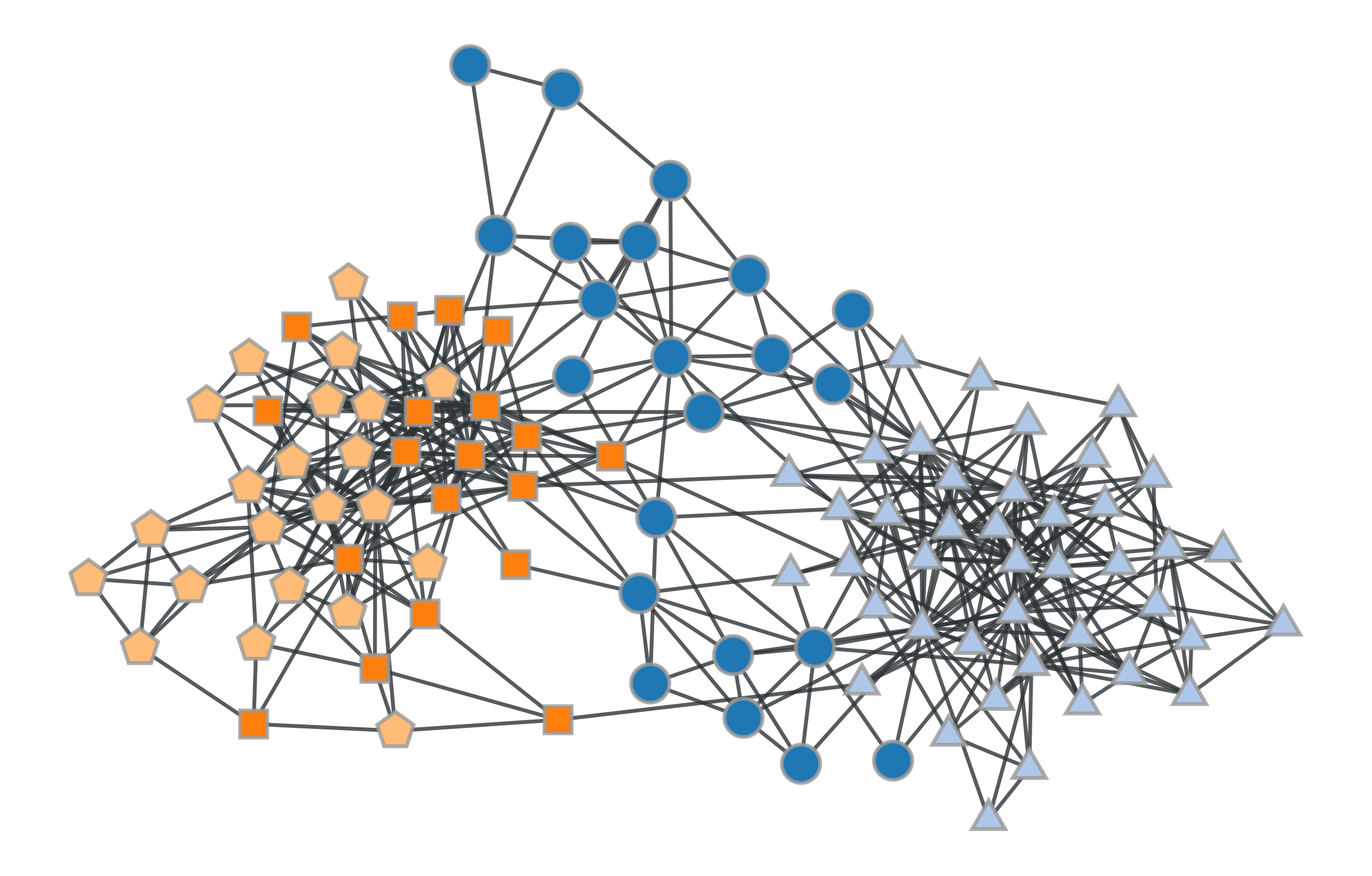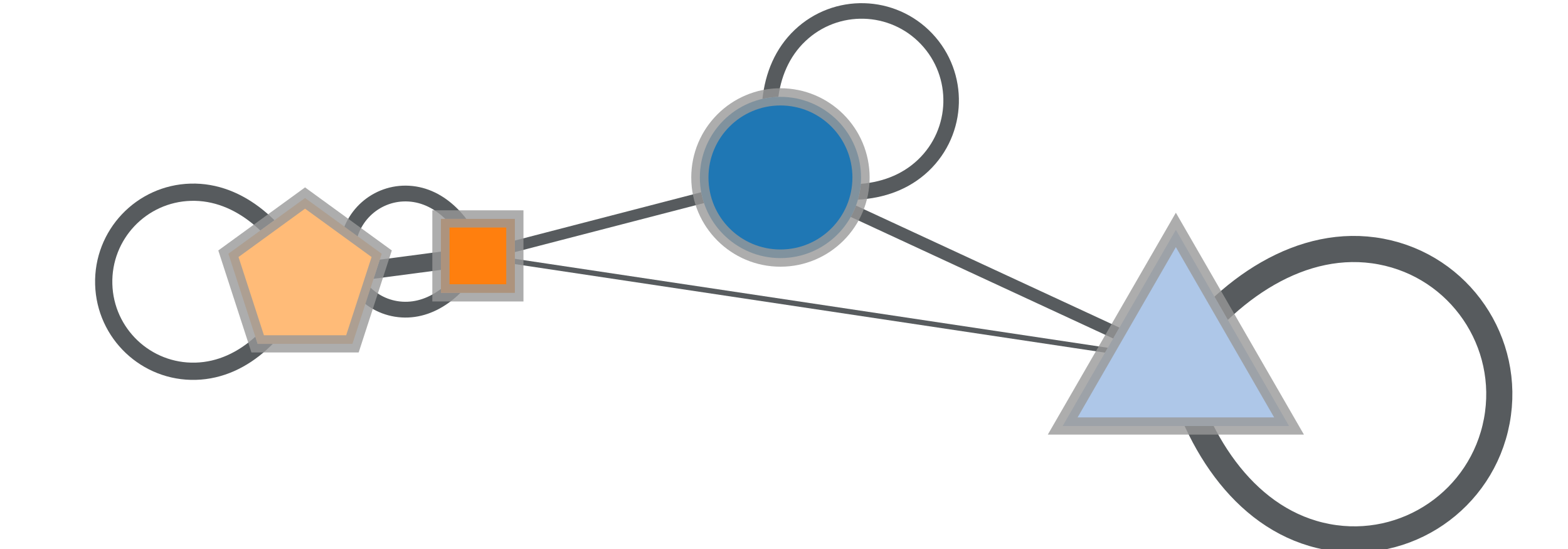# graph_tool.generation.condensation_graph#

graph_tool.generation.condensation_graph(g, prop, vweight=None, eweight=None, avprops=None, aeprops=None, self_loops=False, parallel_edges=False)[source]#

Obtain the condensation graph, where each vertex with the same ‘prop’ value is condensed in one vertex.

Parameters:
gGraph

Graph to be modelled.

propVertexPropertyMap

Vertex property map with the community partition.

vweightVertexPropertyMap (optional, default: None)

Vertex property map with the optional vertex weights.

eweightEdgePropertyMap (optional, default: None)

Edge property map with the optional edge weights.

avpropslist of VertexPropertyMap (optional, default: None)

If provided, the sum of each property map in this list for each vertex in the condensed graph will be computed and returned.

aepropslist of EdgePropertyMap (optional, default: None)

If provided, the sum of each property map in this list for each edge in the condensed graph will be computed and returned.

self_loopsbool (optional, default: False)

If True, self-loops due to intra-block edges are also included in the condensation graph.

parallel_edgesbool (optional, default: False)

If True, parallel edges will be included in the condensation graph, such that the total number of edges will be the same as in the original graph.

Returns:
condensation_graphGraph

The community network

propVertexPropertyMap

The community values.

vcountVertexPropertyMap

A vertex property map with the vertex count for each community.

ecountEdgePropertyMap

An edge property map with the inter-community edge count for each edge.

valist of VertexPropertyMap

A list of vertex property maps with summed values of the properties passed via the avprops parameter.

ealist of EdgePropertyMap

A list of edge property maps with summed values of the properties passed via the avprops parameter.

Notes

Each vertex in the condensation graph represents one community in the original graph (vertices with the same ‘prop’ value), and the edges represent existent edges between vertices of the respective communities in the original graph.

Examples

Let’s first obtain the best block partition with B=5.

>>> g = gt.collection.data["polbooks"]
>>> # fit a SBM
>>> state = gt.BlockState(g)
>>> gt.mcmc_equilibrate(state, wait=1000)
(...)
>>> b = state.get_blocks()
>>> b = gt.perfect_prop_hash([b])
>>> gt.graph_draw(g, pos=g.vp["pos"], vertex_fill_color=b, vertex_shape=b,
...               output="polbooks_blocks_B5.pdf")
<...>


Now we get the condensation graph:

>>> bg, bb, vcount, ecount, avp, aep = \
...     gt.condensation_graph(g, b, avprops=[g.vp["pos"]],
...                           self_loops=True)
>>> pos = avp
>>> for v in bg.vertices():
...     pos[v].a /= vcount[v]
>>> gt.graph_draw(bg, pos=avp, vertex_fill_color=bb, vertex_shape=bb,
...               vertex_size=gt.prop_to_size(vcount, mi=40, ma=100),
...               edge_pen_width=gt.prop_to_size(ecount, mi=2, ma=10),
...               fit_view=.8, output="polbooks_blocks_B5_cond.pdf")
<...>Block partition of a political books network with $$B=5$$.#Condensation graph of the obtained block partition.#